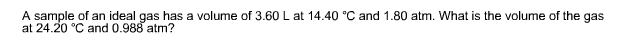# Problem: A sample of an ideal gas has a volume of 3.60 L at 14.40 C and 1.80 atm. What is the volume of the gas at 24.20°C and 0.988 atm?

###### FREE Expert Solution

First, we have to find the moles of gas using the conditions mentioned in the first statement
where P is 1.80 atm, T is 14.40oC, V is 3.60 L and R as 0.0821 (gas constant)
PV=nRT

91% (74 ratings)###### Problem Details

A sample of an ideal gas has a volume of 3.60 L at 14.40 C and 1.80 atm. What is the volume of the gas at 24.20°C and 0.988 atm?